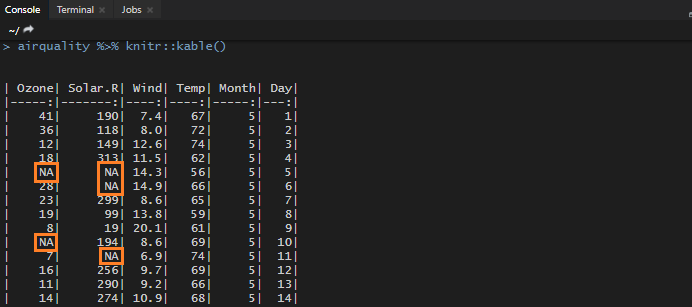# Check if a column has a missing values (NA) in RHere are easy ways how to check if an R data frame column has missing values (NA). It might impact results by using R functions like ifelse, and it is good to know where the NA values might cause a problem.
For example, R data frame that contains NA values is airquality.

In small data frames, you can use view and sorting. If the column contains NA values, they are always at the and of it.If you like to use calculation, then try this. It can check if an R data frame column contains missing values and count them. If the sum of NA values is greater than 0, the column contains them.

```sum(is.na(airquality\$Ozone))
# 37```

To return the names of all R data frame columns and a sum of NA values in them, try this one.

```cbind(
lapply(
lapply(airquality, is.na)
, sum)
)

#        [,1]
#Ozone   37
#Solar.R 7
#Wind    0
#Temp    0
#Month   0
#Day     0```

To get only the names of the R data frame columns that contain missing values, try this one.

```df <- as.data.frame(
cbind(
lapply(
lapply(airquality, is.na), sum)
)
)

rownames(subset(df, df\$V1 != 0))

# "Ozone"   "Solar.R"```

Check out my favorite RStudio tips and tricks. For example, how to quickly view a data frame from R script.